Electric Circuits - Lesson 2 - Electric Current

# Power: Putting Charges to Work

Electric circuits are designed to serve a useful function. The mere movement of charge from terminal to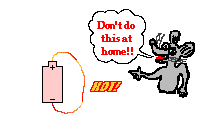terminal is of little use if the electrical energy possessed by the charge is not transformed into another useful form. To equip a circuit with a battery and a wire leading from positive to negative terminal without an electrical device (light bulb, beeper, motor, etc.) would lead to a high rate of charge flow. Such a circuit is referred to as a short circuit. With charge flowing rapidly between terminals, the rate at which energy would be consumed would be high. Such a circuit would heat the wires to a high temperature and drain the battery of its energy rather quickly. When a circuit is equipped with a light bulb, beeper, or motor, the electrical energy supplied to the charge by the battery is transformed into other forms in the electrical device. A light bulb, beeper and motor are generally referred to as a load. In a light bulb, electrical energy is transformed into useful light energy (and some non-useful thermal energy). In a beeper, electrical energy is transformed into sound energy. And in a motor, electrical energy is transformed into mechanical energy.

An electrical circuit is simply an energy transformation tool. Energy is provided to the circuit by an electrochemical cell, battery, generator or other electrical energy source. And energy is delivered by the circuit to the load at the location of the load. The rate at which this energy transformation occurs is of great importance to those who design electrical circuits for useful functions. Power - the rate at which mechanical work is done - was introduced in Unit 5 of the Physics Classroom. Here, we will discuss power in electrical terms; while the context has changed, the essential meaning of the concept of power will remain the same. Power is the rate at which electrical energy is supplied to a circuit or consumed by a load. The electrical energy is supplied to the load by an energy source such as an electrochemical cell. Recall from Lesson 1 that a cell does work upon a charge to move it from the low energy to the high energy terminal. The work done on the charge is equivalent to the electrical potential energy change of the charge. Thus, electrical power, like mechanical power, is the rate at which work is done. Like current, power is a rate quantity. Its mathematical formula is expressed on a per time basis.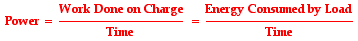Whether the focus is the energy gained by the charge at the energy source or the energy lost by the charge at the load, electrical power refers to the rate at which the charge changes its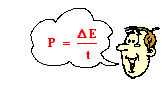energy. In an electrochemical cell (or other energy source), the change is a positive change (i.e., a gain in energy) and at the load, the change is a negative change (i.e., a loss in energy). Thus, power is often referred to as the rate of energy change and its equation is expressed as the energy change per time. Like mechanical power, the unit of electrical power is the watt, abbreviated W. (Quite obviously, it is important that the symbol W as the unit of power not be confused with the symbol W for the quantity of work done upon a charge by the energy source.) A watt of power is equivalent to the delivery of 1 joule of energy every second. In other words:

1 watt = 1 joule / second

When it is observed that a light bulb is rated at 60 watts, then there are 60 joules of energy delivered to the light bulb every second. A 120-watt light bulbs draws 120 joules of energy every second. The ratio of the energy delivered or expended by the device to time is equal to the wattage of the device.

### The kilowatt-hour

Electrical utility companies who provide energy for homes provide a monthly bill charging those homes for the electrical energy that they used. A typical bill can be very complicated with a number of line items indicating charges for various aspects of the utility service. But somewhere on the bill will be a charge for the number of kilowatt-hours of electricity that were consumed. Exactly what is a kilowatt-hour? Is it a unit of power? time? energy? or some other quantity? And when we pay for the electricity that we use, what exactly is it that we are paying for?

A careful inspection of the unit kilowatt-hour reveals the answers to these questions. A kilowatt is a unit of power and an hour is a unit of time. So a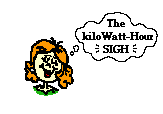kilowatt • hour is a unit of Power • time. If Power = ΔEnergy / time, then Power • time = ΔEnergy. So a unit of power • time is a unit of energy. The kilowatt • hour is a unit of energy. When an electrical utility company charges a household for the electricity that they used, they are charging them for electrical energy. A utility company in the United States is responsible for assuring that the electric potential difference across the two main wires of the house is 110 to 120 volts. And maintaining this difference in potential requires energy.

It is a common misconception that the utility company provides electricity in the form of charge carriers or electrons. The fact is that the mobile electrons that are in the wires of our homes would be there whether there was a utility company or not. The electrons come with the atoms that make up the wires of our household circuits. The utility company simply provides the energy that causes the motion of the charge carriers within the household circuits. And when they charge us for a few hundred kilowatt-hours of electricity, they are providing us with an energy bill.

### Investigate!

The electrical potential difference across the two inserts of a household electrical outlet varies with the country. Use the Household Voltages widget below to find out the household voltage values for various countries (e.g., United States, Canada, Japan, China, South Africa, etc.).

### Calculating Power

The rate at which energy is delivered to a light bulb by a circuit is related to the electric potential difference established across the ends of the circuit (i.e., the voltage rating of the energy source) and the current flowing through the circuit. The relationship between power, current and electric potential difference can be derived by combining the mathematical definitions of power, electric potential difference and current. Power is the rate at which energy is added to or removed from a circuit by a battery or a load. Current is the rate at which charge moves past a point on a circuit. And the electric potential difference across the two ends of a circuit is the potential energy difference per charge between those two points. In equation form, these definitions can be stated as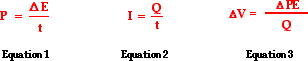Equation 3 above can be rearranged to show that the energy change across the two ends of a circuit is the product of the electric potential difference and the charge - ΔV • Q. Substituting this expression for energy change into Equation 1 will yield the following equation: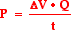In the equation above, there is a Q in the numerator and a t in the denominator. This is simply the current; and as such, the equation can be rewritten as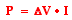The electrical power is simply the product of the electric potential difference and the current. To determine the power of a battery or other energy source (i.e., the rate at which it delivers energy to the circuit), one simply takes the electric potential difference that it establishes across the external circuit and multiplies it by the current in the circuit. To determine the power of an electrical device or a load, one simply takes the electric potential difference across the device (sometimes referred to as the voltage drop) and multiplies it by the current in the device.

### Investigate!

As discussed above, the power delivered to an electrical device in a circuit is related to the current in the device and the electrical potential difference (i.e., voltage) impressed across the device. Use the Electric Power widget below to investigate the effect of varying current and voltage upon the power.

## freestar.config.enabled_slots.push({ placementName: "physicsclassroom_leaderboard_incontent_1", slotId: "physicsclassroom_leaderboard_incontent_3" });

### Check Your Understanding

1. The purpose of every circuit is to supply the energy to operate various electrical devices. These devices are constructed to convert the energy of flowing charge into other forms of energy (e.g., light, thermal, sound, mechanical, etc.). Use complete sentences to describe the energy conversions that occur in the following devices.

a. Windshield wipers on a car

b. Defrosting circuit on a car

c. Hair dryer

2. Determine the ...

a. ... current in a 60-watt bulb plugged into a 120-volt outlet.

b. ... current in a 120-watt bulb plugged into a 120-volt outlet.

c. ... power of a saw that draws 12 amps of current when plugged into a 120-volt outlet.

d. ... power of a toaster that draws 6 amps of current when plugged into a 120-volt outlet.

e. ... current in a 1000-watt microwave when plugged into a 120-volt outlet.

3. Your 60-watt light bulb is plugged into a 110-volt household outlet and left on for 3 hours. The utility company charges you \$0.11 per kiloWatt•hr. Explain how you can calculate the cost of such a mistake.

4. Alfredo deDarke often leaves household appliances on for no good reason (at least according to his parents). The deDarke family pays 10¢/kilowatt-hour (i.e., \$.10/kW•hr) for their electrical energy. Express your understanding of the relationship between power, electrical energy, time, and costs by filling in the table below.

 Power Rating (Watt) Time (hrs) Energy Used (kilowatt-hour) Costs (cents) Costs (\$) 60 Watt Bulb 1 0.060 kW•hr 0.6 ¢ \$0.006 60 Watt Bulb 4 120 Watt Bulb 2 100 Watt Bulb 10 kW-hr 60 Watt Bulb 1000 ¢ \$10 100 60 kW-hr

Next Section:
Jump To Next Lesson:

Follow Us